Updating search results...

# 50 Results

View
Selected filters:
• NC.M1.G-GPE.4Rating
0.0 stars
Overview:

Students learn how to use the Pythagorean theorem and are introduced to an important application of it.

Subject:
Mathematics
Material Type:
Activity/Lab
Provider:
University of Kentucky
Author:
Julie Gibbs and Tara Bennett
02/26/2019Rating
0.0 stars
Overview:

Practice and explanation of using distance formula to calculate area and perimeter of shapes in the coordinate plane.

Subject:
Mathematics
Material Type:
Activity/Lab
Provider:
Math Worksheets Land
02/13/2018Rating
0.0 stars
Overview:

In this Khan Academy activity, students find the area and perimeter of a shape on a coordinate plane.

Subject:
Mathematics
Material Type:
Activity/Lab
Provider:
08/23/2018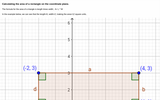Unrestricted Use
CC BY
Rating
2.0 stars
Overview:

Images of rectangle on coordinate plane, one with easily identifiable area, other image with same rectangle rotated. Distance formula shown to calculate same area.

Subject:
Geometry
Material Type:
Interactive
Provider:
Michigan Virtual
07/19/2019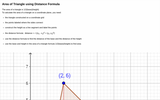Unrestricted Use
CC BY
Rating
1.66666666667 stars
Overview:

Definition of area of triangle, image of triangle on coordinate grid with height constructed. Area of triangle calculated using distance formula.

Subject:
Geometry
Material Type:
Interactive
Provider:
Michigan Virtual
07/19/2019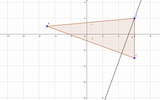Unrestricted Use
CC BY
Rating
0.0 stars
Overview:

This activity promotes critical thinking and experimenting with triangles.

Subject:
Mathematics
Material Type:
Activity/Lab
Author:
VIJAYALAKSHMI SANKARAN
05/27/2020Conditional Remix & Share Permitted
CC BY-NC-SA
Rating
0.0 stars
Overview:

This task explores the real world topic of building light rails. Throughout the implementation of this task the students will learn about the cost of building railways and how to implement them within a budget. This task explores such mathematical concepts of using coordinates to find the distance between points, using coordinates to build polygons and find the area and length of sides, and writing equations of parallel lines.

Subject:
Algebra
Geometry
Material Type:
Activity/Lab
Author:
Carrie Robledo
James O'Neal
03/26/2021Conditional Remix & Share Permitted
CC BY-NC-SA
Rating
4.0 stars
Overview:

CK-12 Foundation's Geometry FlexBook is a clear presentation of the essentials of geometry for the high school student. Topics include: Proof, Congruent Triangles, Quadrilaterals, Similarity, Perimeter & Area, Volume, and Transformations.

Subject:
Mathematics
Material Type:
Textbook
Provider:
CK-12 Foundation
Provider Set:
CK-12 FlexBook
10/06/2009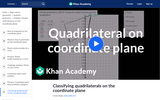Rating
0.0 stars
Overview:

This video classifies shapes as quadrilaterals based on slope.

Subject:
Mathematics
Material Type:
Demonstration
Provider:
02/28/2018Rating
0.0 stars
Overview:

Lesson and practice problems using midpoint, slope and distance formula to prove that figures are certain types of quadrilaterals.

Subject:
Mathematics
Material Type:
Activity/Lab
Provider:
Miami Arts Charter School
02/19/2018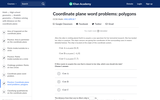Rating
0.0 stars
Overview:

In this Khan Academy activity, students will solve word problems on a coordinate plane using the formulas for distance, area, and perimeter.

Subject:
Mathematics
Material Type:
Activity/Lab
Provider:
08/23/2018Rating
0.0 stars
Overview:

Class activity using distance formula, midpoint formula and cost to plan out a fictional trip

Subject:
Mathematics
Material Type:
Activity/Lab
Provider:
Weebly
11/10/2017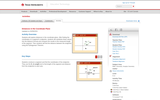Rating
0.0 stars
Overview:

Texas Instruments activity exploring distances in the coordinate plane in order to classify shapes.

Subject:
Mathematics
Material Type:
Activity/Lab
Provider:
Texas Instruments
03/07/2018
RemixConditional Remix & Share Permitted
CC BY-NC
Rating
0.0 stars
Overview:

Students will use distance and perimeter on the coordinate plane to determine which fence company offers the best price for fencing a yard. Depending on the level of the class, students can use the Pythagorean Theorem or the distance formula.

Subject:
Mathematics
Material Type:
Activity/Lab
Assessment
Formative Assessment
Homework/Assignment
Author:
TERESA STEBELTON
06/08/2020
RemixConditional Remix & Share Permitted
CC BY-NC
Rating
1.0 stars
Overview:

Students will use distance and perimeter on the coordinate plane to determine which fence company offers the best price for fencing a yard. Depending on the level of the class, students can use the Pythagorean Theorem or the distance formula.

Subject:
Mathematics
Material Type:
Activity/Lab
Assessment
Formative Assessment
Homework/Assignment
Author:
CARLA JOYNER
11/06/2019
RemixConditional Remix & Share Permitted
CC BY-NC
Rating
0.0 stars
Overview:

Students will use distance and perimeter on the coordinate plane to determine which fence company offers the best price for fencing a yard. Depending on the level of the class, students can use the Pythagorean Theorem or the distance formula.

Subject:
Mathematics
Material Type:
Activity/Lab
Assessment
Formative Assessment
Homework/Assignment
Author:
PATRICIA NORTON
07/10/2020Conditional Remix & Share Permitted
CC BY-NC
Rating
0.0 stars
Overview:

Students will use distance and perimeter on the coordinate place to determine which fence company offers the best price for fencing a yard. Depending on the level of the class, students can use the Pythagorean Theorem or the distance formula.

Subject:
Mathematics
Material Type:
Activity/Lab
Assessment
Formative Assessment
Homework/Assignment
Author:
JOY MCCORMICK
11/06/2019Rating
0.0 stars
Overview:

Editable PPT explaining the distance formula and application of it.

Subject:
Mathematics
Material Type:
Presentation
Provider:
serratellimath.wordpress.com
07/06/2018Conditional Remix & Share Permitted
CC BY-NC-SA
Rating
0.0 stars
Overview:

CK-12's Basic Geometry FlexBook is designed to present students with geometric principles in a simpler, more graphics-oriented course. Students will explore geometry at a slower pace with an emphasis placed on visual aids and approachability.

Subject:
Mathematics
Material Type:
Textbook
Provider:
CK-12 Foundation
Provider Set:
CK-12 FlexBook
Author:
Cifarelli, Victor
Farbizio, Annamaria (Editor)
Gloag, Andrew
Greenberg, Dan
Jordan, Lori
Sconyers, Jim
Zahner, Bill
10/11/2010Conditional Remix & Share Permitted
CC BY-NC-SA
Rating
0.0 stars
Overview:

Given a physical situation (e.g., a room of a certain shape and dimensions, with objects at certain positions and a robot moving across the room), students impose a coordinate system and describe the given in terms of polygonal regions, line segments, and points in the coordinate system.

Subject:
Math 1
Material Type:
Lesson
Author:
EngageNY
02/21/2020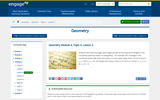Conditional Remix & Share Permitted
CC BY-NC-SA
Rating
0.0 stars
Overview:

Students describe rectangles (with edges parallel to the axes) and triangles in the coordinate plane by means of inequalities. For example, the rectangle in the coordinate plane with lower left vertex (1,2) and upper right vertex (10,15) is {(x,y) l 1 < x < 10 & 2 < y < 15} , the triangle with vertices at (0,0), (1,3), and (2,1) is {(x,y) l x/2 < y < 3x & y < -2x + 5}.

Subject:
Math 1
Material Type:
Lesson
Author:
EngageNY
02/21/2020Conditional Remix & Share Permitted
CC BY-NC-SA
Rating
0.0 stars
Overview:

Given two points in the coordinate plane and a rectangular or triangular region, students determine whether the line through those points meets the region, and if it does, they describe the intersections as a segment and name the coordinates of the endpoints.

Subject:
Math 1
Material Type:
Lesson
Author:
EngageNY
02/21/2020Conditional Remix & Share Permitted
CC BY-NC-SA
Rating
0.0 stars
Overview:

Given a segment in the coordinate plane, students find the segments obtained by rotating the given segment by 90° counterclockwise and clockwise about one endpoint.

Subject:
Math 1
Material Type:
Lesson
Author:
EngageNY
02/21/2020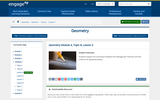Conditional Remix & Share Permitted
CC BY-NC-SA
Rating
0.0 stars
Overview:

Students explain the connection between the Pythagorean Theorem and the criterion for perpendicularity.

Subject:
Math 1
Material Type:
Lesson
Author:
EngageNY
02/21/2020Conditional Remix & Share Permitted
CC BY-NC-SA
Rating
0.0 stars
Overview:

Students recognize parallel and perpendicular lines from slope.
Students create equations for lines satisfying criteria of the kind: “Contains a given point and is parallel/perpendicular to a given line.”

Subject:
Math 1
Material Type:
Lesson
Author:
EngageNY
02/21/2020Conditional Remix & Share Permitted
CC BY-NC-SA
Rating
0.0 stars
Overview:

The challenge of programming robot motion along segments parallel or perpendicular to a given segment leads to an analysis of slopes of parallel and perpendicular lines. Students write equations for parallel, perpendicular, and normal lines. Additionally, students will and study the proportionality of segments formed by diagonals of polygons.

Subject:
Math 1
Material Type:
Lesson
Author:
EngageNY
02/21/2020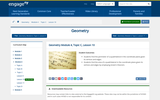Conditional Remix & Share Permitted
CC BY-NC-SA
Rating
0.0 stars
Overview:

Students find the perimeter of a quadrilateral in the coordinate plane given its vertices and edges.
Students find the area of a quadrilateral in the coordinate plane given its vertices and edges by employing Green’s theorem.

Subject:
Math 1
Material Type:
Lesson
Author:
EngageNY
02/21/2020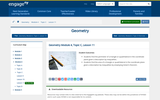Conditional Remix & Share Permitted
CC BY-NC-SA
Rating
0.0 stars
Overview:

Students find the perimeter of a triangle or quadrilateral in the coordinate plane given a description by inequalities.
Students find the area of a triangle or quadrilateral in the coordinate plane given a description by inequalities by employing Green’s theorem.

Subject:
Math 1
Material Type:
Lesson
Provider:
EngageNY
Author:
EngageNY
02/21/2020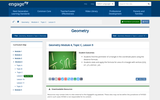Conditional Remix & Share Permitted
CC BY-NC-SA
Rating
0.0 stars
Overview:

Students find the perimeter of a triangle in the coordinate plane using the distance formula.
Students state and apply the formula for area of a triangle with vertices (0,0),(x1, y1), and (x2 , y2).

Subject:
Math 1
Material Type:
Lesson
Author:
EngageNY
02/21/2020Conditional Remix & Share Permitted
CC BY-NC-SA
Rating
0.0 stars
Overview:

Students find midpoints of segments and points that divide segments into 3, 4, or more proportional, equal parts.

Subject:
Math 1
Material Type:
Lesson
Author:
EngageNY
02/21/2020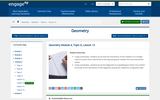Conditional Remix & Share Permitted
CC BY-NC-SA
Rating
0.0 stars
Overview:

Using coordinates, students prove that the intersection of the medians of a triangle meet at a point that is two-thirds of the way along each median from the intersected vertex.
Using coordinates, students prove the diagonals of a parallelogram bisect one another and meet at the intersection of the segments joining the midpoints of opposite sides.

Subject:
Math 1
Material Type:
Lesson
Author:
EngageNY
02/21/2020Conditional Remix & Share Permitted
CC BY-NC-SA
Rating
0.0 stars
Overview:

Students name several points on a line given by a parametric equation and provide the point-slope equation for a line given by a parametric equation.
Students determine whether lines given parametrically are parallel or perpendicular.

Subject:
Math 1
Material Type:
Lesson
Author:
EngageNY
02/21/2020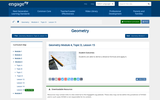Conditional Remix & Share Permitted
CC BY-NC-SA
Rating
0.0 stars
Overview:

Students are able to derive a distance formula and apply it.

Subject:
Math 1
Material Type:
Lesson
Author:
EngageNY
02/21/2020Conditional Remix & Share Permitted
CC BY-NC-SA
Rating
0.0 stars
Overview:

Given a circle, students find the equations of two lines tangent to the circle with specified slopes.
Given a circle and a point outside the circle, students find the equation of the line tangent to the circle from that point.

Subject:
Math 3
Material Type:
Lesson
Author:
EngageNY
02/22/2020Conditional Remix & Share Permitted
CC BY-NC-SA
Rating
4.0 stars
Overview:

This stand-alone module examines the history, applications, and various proofs of the Pythagorean Theorem. The module also includes student activities and exercise problems. The module assumes the reader has a basic geometry background.

Subject:
Mathematics
Material Type:
Textbook
Provider:
CK-12 Foundation
Provider Set:
CK-12 FlexBook
Author:
Sheng, Joy (Editor)
Swenson, Laura
11/08/2010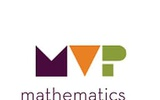Conditional Remix & Share Permitted
CC BY-NC-SA
Rating
0.0 stars
Overview:

The purpose of this task is to develop the distance formula, based upon students’ understanding of the Pythagorean theorem. In the task, students are asked to calculate distances between points using triangles, and then to formalize the process to the distance formula. At the end of the task, students will use the distance formula to find the perimeter of a hexagon.

Subject:
Math 1
Material Type:
Lesson
Author:
The Mathematics Vision Project
03/10/2020Conditional Remix & Share Permitted
CC BY-NC-SA
Rating
0.0 stars
Overview:

Students are given 4-5 coordinate points, that will represent the sheep.  First, they are tasked with creating a fence around the sheep. The students will use the endpoints of each piece of fence to compute the slope, y-intercept, domain and equation of the line. They will then code the rover to draw their lines. Finally, the students can calculate the area/perimeter of their fence and calculate the cost to build the fence. The students will then be challenged to minimize the area, perimeter and cost of building their corral, recalculate the equations of their lines, stating their new slope, y-intercept, and domain.

Subject:
Mathematics
Material Type:
Activity/Lab
Author:
William Allred
Carrie Robledo
05/18/2021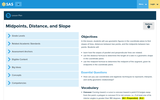Rating
0.0 stars
Overview:

Advanced lesson involving using geometric figures in the coordinate plane to find slopes of lines, distances between two points, and the midpoints between two points. From that point, students can classify polygons based on their definition.

Subject:
Mathematics
Material Type:
Lesson Plan
Provider:
Pennsylvania Department of Education
06/27/2018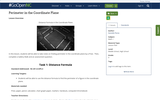Conditional Remix & Share Permitted
CC BY-NC-SA
Rating
0.0 stars
Overview:

Distance Formula in the Coordinate PlaneIn this lesson, students will be able to take notes on finding perimeter in the coordinate plane by a Prezi.  Then, complete a Gallery Walk and an assessment question.

Subject:
Math 1
Material Type:
Lesson Plan
Author:
Danielle Flores
08/08/2019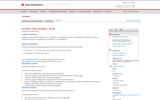Rating
0.0 stars
Overview:

Texas Instruments activity using properties of quadrilaterals then calculating area and perimeter.

Subject:
Mathematics
Material Type:
Activity/Lab
Provider:
Texas Instruments